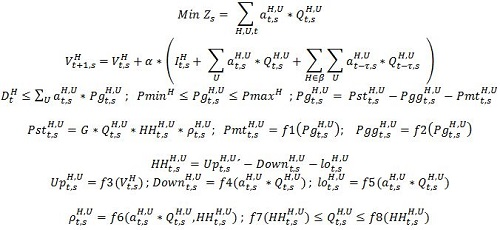EGU21-2903
https://doi.org/10.5194/egusphere-egu21-2903
EGU General Assembly 2021

# A Constructive Heuristic approach for solving Mixed Integer Nonlinear cascade optimization considering an hydrological uncertainty model

Daniel Firmo Kazay and Carlos Roberto Mendonça da Rocha

The quest for a lower operational cost of daily scheduling cascade hydropower generation is subject to imperfections in the representation of reality due to it multiple uncertainties. One way to reduce uncertainties is to improve the representation of the hydrological processes and the operation of the electrical components of the system. A complementary strategy to deal with the difficulty of representing hydrological processes is to move from a deterministic approach to a probabilistic approach considering scenarios. These two proposals usually lead to high-dimensional problems that require Mixed Integer Nonlinear Programming for solving. This work proposes the use of simple Constructive Heuristics to solve this kind of problem guarding the non-linear formulations. The GAMS software was used to represent a hydropower cascade with individualized turbine with multiple inflow scenarios as presented in the formulation bellow. For each scenario s:Z is the objective function. a is a coefficient considered in the proposed Constructive Heuristic. Q is the outflow of the turbine. H is the set of hydropower plants. U is the set of turbines. t is the set of hours considered in the problem. s is the set of considered scenarios. α is a conversion term. τ is the time delay of the hydropower plants. β is the set of hydropower plants located upstream of a hydropower plant. I is the incremental flow. V is the volume of the reservoir. D is the power demand. Pg is power generated per turbine. Pmin and Pmax are the thresholds of power of the turbine. Pst is the net power generated by the turbine. Pgg are the electrical losses of the generator. Pmt are the mechanical losses of the generator. f1, f2, f3, f4, f5, f6, f7 and f8 are functions. HH is the hydraulic net head. UP is the upstream level. Down is the downstream level. Lo are the hydraulic and mechanical losses. ρ is the turbine efficiency curve. The CONOPT solver was selected for it resolution. The  Constructive Heuristic scheme considers a continuous variable “a” to represent if the turbine is opened or closed. After the first resolution of the problem with Nonlinear Programming, the integer part number of turbines for each power plant is fixed. The fractional part is tested for all the possible configurations and the best outcome is chosen. The results of the Constructive Heuristic were compared with other similar works with perfect correspondence. The system was tested for 51 ECMWF precipitation forecast scenarios as input of the hydrologic model MGB (Large Basin Model) with coherent results. The proposal is a feasible approach to reach unique optimal solutions for high-dimensional non-linear problems considering integer variables.

How to cite: Firmo Kazay, D. and Mendonça da Rocha, C. R.: A Constructive Heuristic approach for solving Mixed Integer Nonlinear cascade optimization considering an hydrological uncertainty model, EGU General Assembly 2021, online, 19–30 Apr 2021, EGU21-2903, https://doi.org/10.5194/egusphere-egu21-2903, 2021.